﻿ Exact Solution for Heat Conduction Problem of a Sector of a Hollow Cylinder

### Exact Solution for Heat Conduction Problem of a Sector of a Hollow Cylinder

Saeed Rafee NekooOPEN ACCESSPEER-REVIEWED

## Exact Solution for Heat Conduction Problem of a Sector of a Hollow Cylinder

Saeed Rafee NekooSchool of mechanical engineering, Iran University of Science and Technology (IUST), Tehran, Iran

### Abstract

In this article, the heat conduction problem of a sector of a finite hollow cylinder is studied as an exact solution approach. The governing equations are in the form of non-homogeneous partial differential equation (PDE) with non-homogeneous boundary conditions. In order to solve the PDE equation, generalized finite Hankel, periodic Fourier, Fourier and Laplace transforms are applied. Three different boundary conditions as case studies for simulations are presented and verified with the result which extracted from finite element method. The results are shown that this approach is suitable and systematic for solving heat conduction problems in cylindrical coordinate.

### At a glance: Figures

123456
Prev Next

• Nekoo, Saeed Rafee. "Exact Solution for Heat Conduction Problem of a Sector of a Hollow Cylinder." American Journal of Mechanical Engineering 1.2 (2013): 50-57.
• Nekoo, S. R. (2013). Exact Solution for Heat Conduction Problem of a Sector of a Hollow Cylinder. American Journal of Mechanical Engineering, 1(2), 50-57.
• Nekoo, Saeed Rafee. "Exact Solution for Heat Conduction Problem of a Sector of a Hollow Cylinder." American Journal of Mechanical Engineering 1, no. 2 (2013): 50-57.

 Import into BibTeX Import into EndNote Import into RefMan Import into RefWorks

### 1. Introduction

Measuring and finding the distribution and variation of heat conduction directly is not an easy work to do and not possible for some cases and that is one of the significant purposes of presenting different methods for solving heat conduction problems. Hoshan presented a triple integral equation method for solving heat conduction equation . A new kind of triple integral was employed to find a solution of non-stationary heat equation in an axis-symmetric cylindrical coordinates under mixed boundary of the first and second kind conditions. Kayhani et al. introduced a general analytical solution for heat conduction in cylindrical multilayer composite laminates . In the article, the direction of fiber was able to change between the layers. The boundary condition was considered linear and the method was introduced suitable for boundary conditions consisting of conduction, convection and radiation. Cossali expressed an analytical solution of the steady periodic heat conduction in a solid homogenous finite cylinder via Fourier transform, with the sole restriction of uniformity on the lateral surface and radial symmetry on the bases . A harmonic heating, as an example, introduced with simulation results. Matysiak et al. expressed the problem of transient heat conduction in a from-time-to-time arranged in layers consisting of a large number of interchanging concentric cylinders . The cylinders have a great quantity of circular homogenous isotropic rigid sectors. Sommers and Jacobi presented an exact solution to steady heat conduction in a two-dimensional hollow on a one-dimensional fin . The fin efficiency of a high thermal conductivity was discussed. The exact solution was obtained by separation of variables method. Jabbari et al. explained an analytical solution to a problem of one-dimensional moving heat source in a hollow FGM cylinder . Mechanical and thermal stresses were considered and the material in the case, varied continuously across the thickness. The method of solution was direct and used Bessel function. Atefi and Talaee presented non-Fourier heat conduction in a finite hollow cylinder with periodic surface heat flux . The material assumed homogenous and isotropic temperature-independent thermal properties. The separation of variable method was applied for solving the time-independent boundary condition and the Duhamel integral was used to apply for time dependent part.

In this paper, in order to solve the problem as an exact method, generalized finite Hankel transform is used. Hankel is a transformation for solving problems consisting of cylindrical coordinates, but not the hollow one. Eldabe et al. introduced an extension of the finite Hankel transform which was capable of solving problems in hollow cylindrical coordinates, heat equation or wave with mixed boundary values . Povstenko expressed the radial diffusion in a cylinder via Laplace and Hankel transform . Akhtar presented exact solutions for rotational flow of a generalized Maxwell fluid between two circular cylinders . In order to find the exact solution Laplace and finite Hankel transforms were employed. Fetecau et al. introduced exact solutions for the flow of a viscoelastic fluid induced by a circular cylinder subject to a time dependent shear stress via finite Hankel transform . Yu et al. expressed general temperature computational method of linear heat conduction for multilayer cylinder .

### 2. Governing Equations

The general equation of heat conduction problem of a circle sector of a hollow cylinder is defined as following :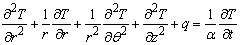(1)

where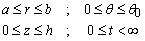are the limits of the domains and T is a function of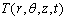,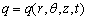is a heat source for the problem, and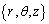demonstrate the cylindrical coordinates that are shown in Figure 1. t stands for time,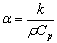represents thermal diffusivity in which k stands for thermal conductivity,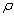represents density and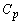is the specific heat.

Boundary conditions are chosen as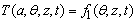(2)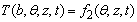(3)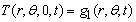(4)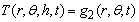(5)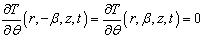(6)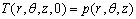(7)

where a is the inner radius of the cylinder and b is the outer one, h is the height of the cylinder and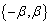are the angular limits of the sector.

### 3. Derivation of the Exact Solution

In order to solve the PDE, four transformations should be applied. The first transformation is periodic Fourier transform which applies in rotational direction and changes the domain of θ to m and transforms Equation (1) to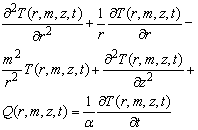(8)

in which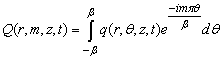(9)

The second and third transformations change the domain of z to ω and t to s, Fourier sine and Laplace transform, then the Equation (8) can be rewritten in the form of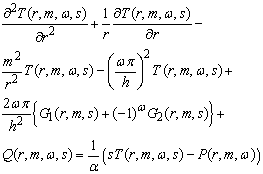(10)

where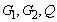and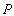are the transformed form of boundary and initial conditions. Finally, the last transformation, generalized finite Hankel transform, changes the domain of r to domain of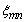. This transformation is expressed as bellow :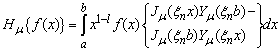(11)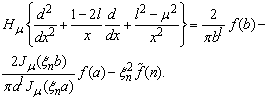(12)

So according to Equation (11), with considering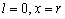and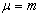, Equation (10) can be written as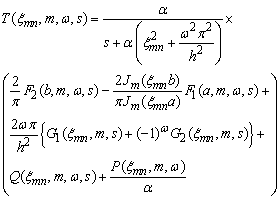(13)

where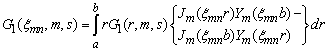(14)

and similarly for others in which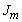is the Bessel function of the first kind and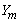is the Bessel function of the second kind. Then inverse Laplace, inverse periodic Fourier and inverse Fourier sine transforms need to be used to shape the answer as Equation (15), in the following: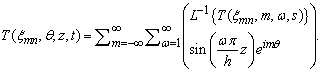(15)

Inverse generalized finite Hankel transform is presented as in the following :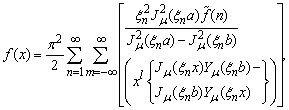(16)

and the exact solution of the problem is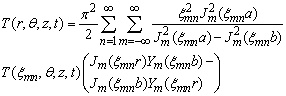(17)

in whichare the roots of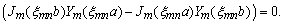(18)

### 4. Case Studies

4.1. Axis Symmetric Conditions

In the first case, the problem is considered axis-symmetric, so the term of second derivative respect to θ equals to zero and the problem is independent from θ. The boundary conditions are considered as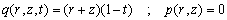(19)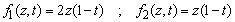(20)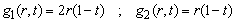(21)

The heat source in Equation (19) changes during the time which is a function of z, t, r. The boundary conditions, inside and outside of the sector of hollow cylinder are considered as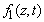and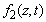in Equation (20) dependent on time and z, and the initial condition is regarded zero, Equation (19). The parameters of the PDE are considered as Table 1:

#### Table 1. Parameters of the problemDownload asPowerPoint Slide

Veiw figureView current table in a new window

Applying the conditions on the PDE equation and solving by the proposed method, following results are obtained. In Figure 2 the distribution of temperature using finite element method is shown at t=0.01sec The details and comparisons between two methods are shown at t=0.01sec and z={0.25,0.5,0.75}m in Figure 3, Figure 4, Figure 5. In Figure 6, the variations of temperature are shown at t={0.01,0.5,1}sec, in different sections, z={0.25,0.5,0.75}m with respect. The temperature field in the bottom and the top of the sector cylinder are zero and they are not presented in Figure 6. According to the comparisons between generalized finite Hankel transform method and finite element method, this approach manages the problem in an appropriate way. The jump in FEM results is due to automatic meshing of the model via ANSYS software.

Since the boundary conditions are continuous functions, it is expected to attain smooth results. The negligible difference between FEM and exact solution is due to numerical calculations in ANSYS software.

Figure 6. The variations of temperature for case 1; (a) t=0.01s, z=0.25m, (b) t=0.5s, z=0.25m, (c) t=1s, z=0.25m, (d) t=0.01s, z=0.5m, (e) t=0.5s, z=0.5m, (f) t=1s, z=0.5m, (g) t=0.01s, z=0.75m, (h) t=0.5s, z=0.75m, (i) t=1s, z=0.75m
4.2. Asymmetric Conditions

In the second case, the conditions are considered dependent on{θ, z, t}. There is a point to explain in this case so the conditions are chosen as simply as possible in the following.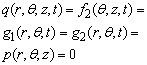(22)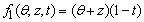(23)

Applying the conditions on the PDE equation and solving, the closed form answer is obtained in the following.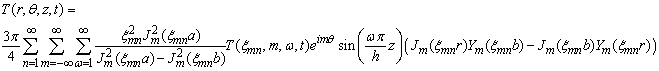(24)

in which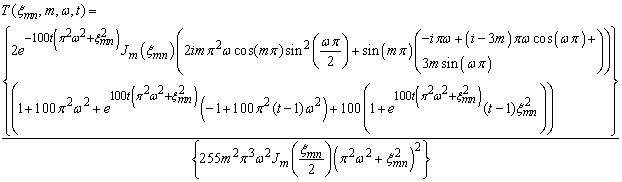(25)

The following results are attained due to simulation. In Figure 7 the distribution of temperature via the finite element method is shown, at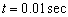. The details of changes in temperature field can be demonstrated in different sections, Figures 8, Figures 9, Figures 10, Figures 11 show the details of sections at θ={20,30,40,50} (degree) at t=0.01sec and z=0.25m, with respect. In Figure 12, the variation of temperature are shown at t={0.01,0.51}sec, in different sections, z={0.25,0.5,0.75}m, with respect.

According to boundary condition in Equation (23), the temperature value at r=1 is zero which can be seen in Figures 8,9,10,11. The temperature at r=0.5 increases by changing the θ between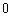to π/3.

In Figure 13 it is shown that the temperature is increased near the inside radius of the one section of the sector cylinder in the range of zero to β and it is decreased in the range of zero to -β. According to Equation (23) the temperature cannot be negative, like Figure 7 which is true and the range changes between zero to β. The reason of this difference is that the periodic Fourier transform is applied between -β to β. So, in the half of the cylinder as they are presented in Figures 8,9,10,11, the results are verified. This problem is one of the limitations of this method. The wave shape of the results which are attained in exact method, caused due to considering m between -10 to 10 and n between 1 to 20. For a better and closer answer the values of m, n ought to be chosen in a wider range.

Figure 12. The variations of temperature for case 2; (a) t=0.01s, z=0.25m, (b) t=0.5s, z=0.25m, (c) t=1s, z=0.25m, (d) t=0.01s, z=0.5m, (e) t=0.5s, z=0.5m, (f) t=1s, z=0.5m, (g) t=0.01s, z=0.75m, (h) t=0.5s, z=0.75m, (i) t=1s, z=0.75m
Figure 17. The variations of temperature for case 3; (a) t=0.01s, z=0.25m, (b) t=0.5s, z=0.25m, (c) t=1s, z=0.25m, (d) t=0.01s, z=0.5m, (e) t=0.5s, z=0.5m, (f) t=1s, z=0.5m, (g) t=0.01s, z=0.75m, (h) t=0.5s, z=0.75m, (i) t=1s, z=0.75m
4.3. General Case

In the third simulation a general case is considered. The boundary and initial conditions are presented as.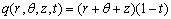(26)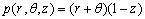(27)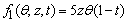(28)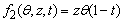(29)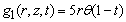(30)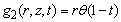(31)

and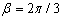rad. Figures 14-16 show the results of solving the PDE in a general case in different sections, z={0.25,0.5,0.75}, with respect. In Figure 17 temperature field in different sections of the cylinder are presented.

The results of the general case are obtained with considering m between -5 to 5 and n between 1 to 5. The results can be improved by expanding the range of series which increases the time of solving.

### 5. Conclusions

In this article, the generalized finite Hankel transform was employed to solve the heat conduction problem of a sector of a hollow cylinder with non-homogeneous boundary conditions. The details of the transformations were explained and three case studies were shown. The finite element method was used for verifying the results. The exact solution method, provided in this paper, is in a systematic form and formulations and applicable for any initial and boundary conditions. The accuracy of the results can be enhanced by expanding the range of the terms in series. The FEM on the other hand is a good approach for obtaining the numerical results for these kinds of systems; however meshing and applying the initial and boundary conditions are somehow more difficult than the exact solution approach. The comparisons of the two methods were verified each other and show that this method, as an exact solution technique, is appropriate for these problems.

### References

  Hoshan N.A., The Triple Integral Equations Method for Solving Heat Conduction Equation, Journal of Engineering, Thermophysics, 18 (3), 2009, 258-262.In article  Kayhani M.H., Norouzi M., Delouei A.A., A General Analytical Solution for Heat Conduction in Cylindrical Multilayer Composite Laminates, Int. Journal of Thermal Sciences 52, 2011, 73-82.In article CrossRef  Cossali G.E., Periodic Heat Conduction in a Solid Homogeneous Finite Cylinder, Int. J. of Thermal Sciences 48, 2009, 722-732.In article CrossRef  Matysiak S.J., Pauk V.J., Yevtushenko A.A., On Applications of the Microlocal Parameter Method in Modelling of Temperature Distributions in Composite Cylinders, Archive of Applied Mechanics 68, 1998, 297-307.In article CrossRef  Sommers A.D., Jacobi A.M., An Exact Solution to Steady Heat Conduction in a Two-Dimensional Annulus on a One-Dimensional Fin: Application to Frosted Heat Exchangers with Round Tubes, Journal of Heat Transfer 128, 2006, 397-404.In article CrossRef  Jabbari M., Mohazzab A.H., Bahtui A., One-Dimensional Moving Heat Source in a Hollow FGM Cylinder, J. of Pressure Vessel Technology 131, Transactions of the ASME, 021202, 2009.In article  Talaee M.R., Atefi G., Non-Fourier Heat Conduction in a Finite Hollow Cylinder with Periodic Surface Heat Flux, Archive of Applied Mechanics 81, 2011, 1793-1806.In article CrossRef  Eldabe N.T., El-Shahed M., Shawkey M., An Extension of the Finite Hankel Transform, Applied Mathematics and Computation 151, 2004, 713-717.In article CrossRef  Povstenko Y.Z., Fractional Radial Diffusion in a Cylinder, Journal of Molecular Liquids, 137, 2008, 46-50.In article CrossRef  Akhtar W., Siddique I., Sohail A., Exact Solutions for the Rotational Flow of a Generalized Maxwell Fluid Between two Circular Cylinders, Commun Nonlinear Sci Numer Simulat 16, 2011, 2788-2795.In article CrossRef  Fetecau C., Mahmood A., Jamil M., Exact Solutions for the Flow of a Viscoelastic Fluid Induced by a Circular Cylinder Subject to a Time Dependent Shear Stress, Commun Nonlinear Sci Numer Simulat 15, 2010, 3931-3938.In article CrossRef  Yu C.L., Shen L.Y., Man F., Rani X., General Temperature Computational Method of Linear Heat Conduction Multilayer Cylinder, International Journal of Iron and Steel Research 17, 2010, 33-37.In article CrossRef  M.N. Ozisik, Heat Conduction, Second Edition, Wiley & Sons, Inc., 1993.In article